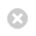Close

UCL Great Ormond Street Institute of Child Health

HomeGreat Ormond Street Institute of Child Health# Chapter 4: Quantifying Differences and Associations

The previous chapter considered summary statistics for a single sample. In this section we look at ways of quantifying the differences between two groups and also looking at associations between different variables measured on the same individual.

The research study should be designed to answer a specific research question - usually to do with quantifying differences between groups (healthy/diseased or treated/controls)

Quantification depends on whether the variable is categorical or numeric. In each case we consider 2 groups (such as healthy individuals vs those with disease or treated vs. untreated) and there is a single categorical or numeric outcome to compare, for example, reaction to a stimulus (positive or negative), or blood pressure.

Quantifying Categorical Differences

We here consider a single binary outcome. The results are easily extended to ordinal or nominal outcomes and a comparison of the numbers falling into one of the categories.

Firstly we should present the results in a contingency (or frequency) table. In the case of a binary variable compared between two groups this is known as a 2x2 frequency table.

For example, suppose we wish to compare how many infants suffer colic between healthy babies and those with cystic fibrosis. If we study 360 healthy babies and 300 with cystic fibrosis and 68 and 92 of each group respectively have colic. These results can be displayed in the 2x2 table thus:The proportion with colic in the healthy group is 68/360 = 0.189, which is a percentage 18.9%. We might also say that the rate of colic in this group is 0.189 or 18.9 per 100.

The odds of colic are 68/292 = 0.232.

The proportion with colic in the CF group is 92/300 = 0.307, which is a percentage 30.7%. We might also say that the rate of colic in this group is 0.307 or 30.7 per100.

The odds of colic are 92/208 = 0.442

So, colic occurs more often in the CF group. There are various ways to quantify the difference in the healthy and CF babies in relation to colic occurrence:

(i) The difference in proportions: 0.307-0.189 = 0.118 more of the CF group have colic

(ii) The difference in percentages: 11.8% more of the CF group have colic

(iii) The relative risk (RR) is the relative difference in risks: 0.307/0.189 = 1.624

This means that those with CF are 1.624 times more likely to have colic - an increase of 62.4%.

Alternatively, 0.189/0.307 = 0.616. When the RR is expressed this way round the interpretation is that the healthy children are 0.616 times as likely to have colic as a child with CF i.e., they are 38.4% less likely.

(iv) The odds ratio (OR) is the ratio of the odds: 0.442/0.232 = 1.905 or 0.232/0.442 = 0.525

I.e., the odds of colic in the CF group are 1.905 times those in the healthy group; in the healthy group they are just over half (0.525) the odds in the CF group.

So, there are various ways of expressing the difference. None is any more valid than the others, they highlight different aspects. Those with CF are 11.8% more likely to have colic or have a 62.4% higher risk, their odds are increased by 90.5%.

It is easy to see why we need to be careful and clear about which measure is being used!

Quantifying Numeric Differences

If we have a numeric variable to compare between groups then we should first consider the distribution within each group. We can do this using summary statistics and a dot-plot of the data (or bar-charts within each group).

Example:

Information was collected from 147 children with Sickle Cell disease. We may wish to compare outcomes between boys and girls. The outcomes we will consider are:

(i) Mean oxygen saturation

(ii) Haemoglobin F at baseline

(iii) Mean haemoglobin between 11 & 25 months of age

The data is summarized in the following table and plots:Oxygen saturation is downwardly skew (hence the means are lower than the medians), haemoglobin F is upwardly skew (hence means higher than medians) and mean haemoglobin between 11 & 25 months is approximately normally distributed (means and median approximately equal).

The differences between boys and girls are therefore best quantified as the difference in medians for the first two measurements and the difference in means for haemoglobin between 11 & 25 months. These are:

(i) Oxygen saturation: 96.14 - 96.055 = 0.085

(ii) Haemoglobin F: 7.3 - 6.7 = 0.6

(iii) Haemoglobin 11-25 months: 9.279-8.842 = 0.437

So, the girls have median levels of oxygen saturation and haemoglobin F that are 0.085 and 0.6 higher than the boys. Their mean 11-25 month haemoglobin is 0.437 higher.

Quantifying Associations

Sometimes we wish to identify patterns between two or more variables measured on the same individual; for example, between the two haemoglobin measurements. We can plot these to see what the association looks like:A measure of association is given by the correlation coefficient.

The Pearson correlation, denoted by r, takes values between -1 and 1 and quantifies how close the points lie to a straight line:

Correlation coefficients lie between -1 and +1.

The Pearson correlation coefficient, r, takes values of +1 or -1 only if the relationship is perfectly linear:Positive values indicate that as one variable increases so does the other; negative values indicate that as one variable increases the other decreases.

The closer the coefficient is to zero, the less the linear association:If r=0, there is no linear association between the variables. This however does not necessarily mean that there is NO association between the variables.The correlation between the two haemoglobin measurements is 0.236 which indicates some (not major) degree of linear association.

The relationship between one variable and others jointly can be further quantified using regression analysis. However, this is beyond the scope of this course.